### Score

New Quiz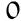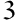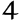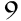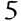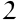Players

Addition is one of the basic mathematical operations, symbolized by the “+” sign. It consists in calculating the sum of two or more numbers. For example, in the addition 3 + 4, “3” and “4” are called the terms, and “7” is the sum.

Addition is an ancient operation, used by the most primitive civilizations to count and exchange goods. Numerical systems and methods of addition have evolved over time, becoming increasingly sophisticated with the invention of written numbers, the introduction of the zero and the development of mathematics.

Addition is fundamental to mental arithmetic. It is often the first mathematical operation taught to children, as it forms the basis for learning other mathematical concepts, such as subtraction and multiplication. Mastering addition is essential for developing logical thinking and solving everyday problems, such as calculating expenses or measuring time.

Let’s take a simple example: if you have three apples and add two more, you’ll get five apples in all, which can be represented as 3 + 2 = 5. This type of simple addition is a great way to start understanding the concept of addition and develop mental arithmetic skills.

When venturing into the field of mathematics, it’s essential to understand basic terms such as “term” and “sum”. These concepts may seem simple, but they are fundamental to mastering more complex mathematical operations.

In mathematics, a term generally refers to a single mathematical element, be it a single number, a variable or the product of a number and a variable. For example, in the expression 3x + 4, “3x” and “4” are the terms.

The sum, on the other hand, is the result of adding two or more terms. For example, in the addition 3 + 4, “7” is the sum of the terms “3” and “4”.

While the term refers to a part of a mathematical expression or equation, often a number or variable, the sum is the total result of adding two or more terms. A term is a component of the addition operation, while the sum is the product of this operation.

To illustrate our point, let’s consider the expression 5 + 9 = 14. Here, “5” and “9” are the terms of the addition, and “14” is the sum. The terms are the elements we add together, and the sum is the result of this addition.

By understanding the distinction between a term and a sum, we can construct and solve more complex equations and better understand mathematical principles.

Mental addition is a key life skill, making everyday tasks such as shopping and budgeting easier. Here are some strategies for quickly calculating an addition.

• Grouping numbers: Grouping similar or complementary numbers can greatly simplify addition. For example, adding 5 and 5 is faster than adding 5 and 8, because we immediately know that 5 + 5 = 10.
• Using the associative property: The associative property states that the order in which we add numbers doesn’t change the sum, which can be useful for rearranging terms to simplify the calculation. For example: ( (2 + 3) + 4 = 2 + (3 + 4) ).
• Using the commutative property: The commutative property of addition allows you to change the order of terms without changing the sum, ( a + b = b + a ). This property makes it possible to rearrange numbers to find the sum more quickly.

### Applying mental arithmetic techniques

Suppose you need to quickly add 7, 3, 4 and 6. Using the associative property, rearrange 7 and 3 to get 10, and 4 and 6 to get 10, then add the two sums to get 20 quickly.

### How to use these techniques in everyday life

• Shopping: When shopping, group prices of similar items and use the associative property to quickly add up the subtotals.
• Budgeting: When budgeting, group your expenses by category, then use the commutative and associative properties to quickly calculate your total expenses.

### Tips for improving speed and accuracy in mental arithmetic

• Regular practice: Regular practice of mental arithmetic strengthens memory and improves speed and accuracy.
• Play number games: Games like Sudoku or card games can be a fun way to improve your mental arithmetic skills.
• Use math apps: Many apps offer mental arithmetic exercises to improve speed and accuracy.

By mastering these techniques and practicing regularly, you’ll be able to perform mental additions quickly and accurately, making many everyday tasks easier.